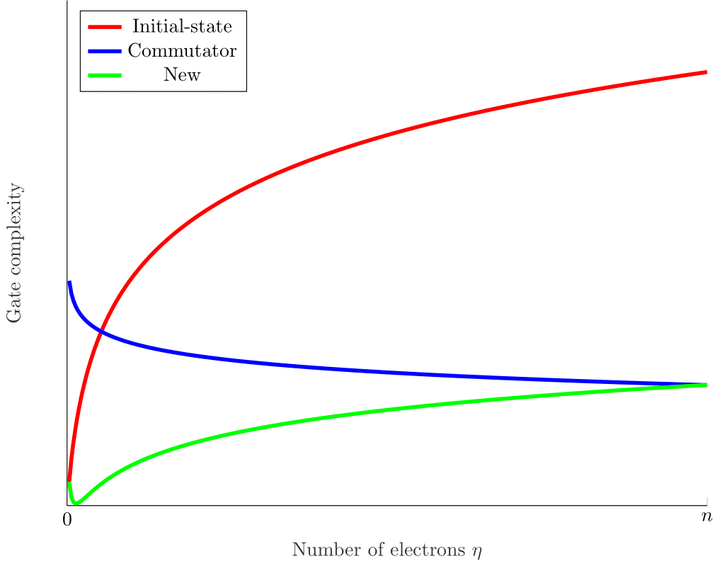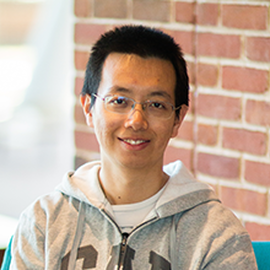# Nearly tight Trotterization of interacting electronsImage credit: Hsin-Yuan Huang

### Abstract

We consider simulating quantum systems on digital quantum computers. We show that the performance of quantum simulation can be improved by simultaneously exploiting commutativity of the target Hamiltonian, sparsity of interactions, and prior knowledge of the initial state. We achieve this using Trotterization for a class of interacting electrons that encompasses various physical systems, including the plane-wave-basis electronic structure and the Fermi-Hubbard model. We estimate the simulation error by taking the transition amplitude of nested commutators of the Hamiltonian terms within the $\eta$-electron manifold. We develop multiple techniques for bounding the transition amplitude and expectation of general fermionic operators, which may be of independent interest. We show that it suffices to use $\left(\frac{n^{5/3}}{\eta^{2/3}}+n^{4/3}\eta^{2/3}\right)n^{o(1)}$ gates to simulate electronic structure in the plane-wave basis with $n$ spin orbitals and $\eta$ electrons, improving the best previous result in second quantization up to a negligible factor while outperforming the first-quantized simulation when $n=\eta^{2-o(1)}$. We also obtain an improvement for simulating the Fermi-Hubbard model. We construct concrete examples for which our bounds are almost saturated, giving a nearly tight Trotterization of interacting electrons.

Type
Publication
Quantum 5, 495 (2021)##### Yuan Su
###### Senior Researcher

I work on quantum algorithms for simulating Hamiltonian dynamics. I am particularly interested in the design, analysis, implementation, and application of quantum simulation.